# NCERT Solution Exercise 13.2 Class 10

Note:

• In this Exercise we will study the Volume of a Combination of Solids
• Unless the stated otherwise, take π = 22/7

Q,1 A solid is in the shape of a cone standing on a hemisphere with both their radii being equal to 1 cm and the height of the cone is equal to its radius. Find the volume the Solid in terms of π

Solution: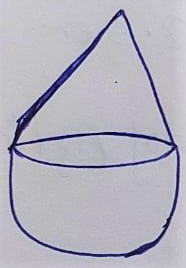r = 1 cm

Height of cone (h)= Radius = 1 cm

Volume of the Solid = Volume of the cone + Volume of hemisphere

⇒ Volume of the Solid = 1/3 ×πr²h + 2/3 × πr³

⇒ Volume of the Solid = 1/3 ×πr²( h + 2 r )

⇒ Volume of the Solid = 1/3 ×π(1)²( 1 + 2 ×1 )

⇒ Volume of the Solid = 1/3 ×π( 3 )

⇒ Volume of the Solid = π

Q.2 Rachel, an engineering student, was asked to make a model shaped like a cylinder with two cones attached at its two ends by using a thin aluminum sheet. The diameter of the model is 3 cm and its length is 12 cm. If each cone has a height of 2 cm. Find the volume of air contained in the model that Rachel made. ( Assume the outer and inner dimensions of the model to be nearly the same)

Given:

Diameter = 3 cm

Height of model = 12 cm

To Find:

Volume of air contained in the model =?

Solution: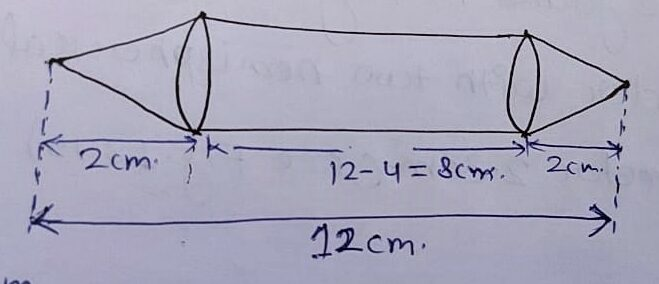Height of cylindrical part = 12 – 4 = 8 cm

The Volume of air contained in the model = 2×volume of cone + volume of cylinder

⇒ The Volume of air contained in the model = 2×1/3 ×πr²h + πr²h

⇒ The Volume of air contained in the model = πr²( 2/3 ×h + h)

⇒ The Volume of air contained in the model = 22/7 × 3/2 × 3/2 ( 2/3 ×2 + 8)

⇒ The Volume of air contained in the model =(11×3×3)/ 7×2 ( 4/3  + 8)

⇒ The Volume of air contained in the model = 11×3×3/ 7×2 ( 4+24)/3

⇒ The Volume of air contained in the model = 99/14 ×( 28)/3

⇒ The Volume of air contained in the model = 66 cm³

Q. 3 A Gulab jamun, contains sugar syrup up to about 30% of its volume .Find approximately how much syrup would be found in 25 Gulab jamuns, each shaped like a cylinder with two hemispherical ends with length 5 cm and diameter 2.8 cm (see fig. 13.15)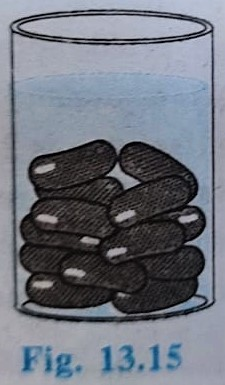Given:

Each Gulab jamun:

Contains sugar syrup = 30% of its volume

Number of Gulab jamuns = 45

Each Length = 5 cm

Diameter = 2.8 cm

To Find:

Amount of sugar syrup in 45 Gulab jamuns =?

Solution: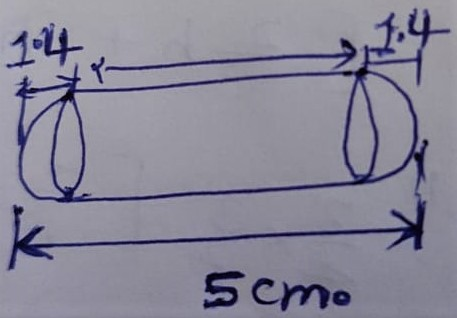Height of cylindrical part = 5 – 2.8 = 2.2 cm

Volume of each Gulab jamun = 2 ×Volume of hemispherical part + Volume of cylindrical part

⇒ Volume of each Gulab jamun = 2 × 2/3 × πr³ + πr²h

⇒ Volume of each Gulab jamun = πr²(2×2/3 × r + h)

⇒ Volume of each Gulab jamun = 22/7 × 1.4×1.4×(4/3 × 1.4 + 2.2)

⇒ Volume of each Gulab jamun = 22/7 × 1.4×1.4×(4/3 × 1.4 + 2.2)

⇒ Volume of each Gulab jamun = 4.4×1.4×(5.6/3+ 2.2)

⇒ Volume of each Gulab jamun = 4.4×1.4×(5.6+ 6.6)/3

⇒ Volume of each Gulab jamun = 4.4×1.4× 12.2/3

Volume of 45 Gulab jamun = 45× volume of each Gulab jamun

⇒ Volume of 45 Gulab jamun = 45× 4.4×1.4× 12.2/3

⇒ Volume of 45 Gulab jamun = 9× 4.4×1.4× 12.2

⇒ Volume of 45 Gulab jamun = 338.1840 cm³ ≈ 338 (approx.)

Hence Amount of sugar syrup in 45 Gulab jamuns = 338 cm³

Q.4 A pen stand made of wood is in the shape of a cuboid with four conical depressions to hold pens. The dimensions of the cuboid are 15 cm by 10 cm by 3.5 cm. The radius of each of the depressions is 0.5 cm and the depth is 1.4 cm Find the volume of wood in the entire stand. ( see fig. 13.16 )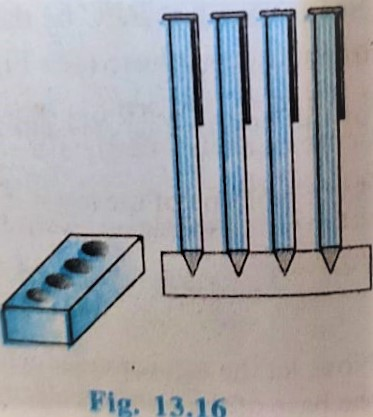Solution:

Dimension of Cuboid:

l = 15 cm

b = 10 cm

h = 3.5 cm

Depth (h) = 1.4 cm

The Volume of wood in the entire Stand = Volume of cuboid – 4×Volume of cone

⇒ The Volume of wood in the entire Stand = l × b × h – 4×1/3 × πr²h

⇒ The Volume of wood in the entire Stand = 15 × 10 × 3.5 – 4×1/3 × 22/7 × 0.5×0.5 ×1.4

⇒ The Volume of wood in the entire Stand = 525.0 – 4×1/3 × 22/7 × 5/10 × 5/10 ×14/10

⇒ The Volume of wood in the entire Stand = 525.0 – 4×11 / 3×10

⇒ The Volume of wood in the entire Stand = 525.0 – 44 / 3×10

⇒ The Volume of wood in the entire Stand = 525.0 – 14.66 / 10

⇒ The Volume of wood in the entire Stand = 525.0 – 1.466

⇒ The Volume of wood in the entire Stand = 523.53 cm³

Q.5 A vessel is in the form of an inverted cone. It’s height is 8 cm and the radius of its top which is open, is 5 cm. It is filled with water up to the brim. When lead shots, each of which is a sphere of radius 0.5 cm are dropped into the vessel one fourth of the water flows out Find the number of lead shots dropped in the vessel.

Given:

Dimension of vessel:

Height (h) = 8 cm

To Find:

The number of lead-shots dropped in the vessel = ?

Solution: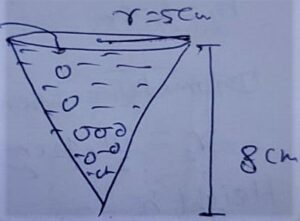Let number of lead shots = x

Volume of total Number of lead-shots dropped in the vessel = 1/4 ×volume of water

⇒ x ×4/3 × πr² = 1/4 × 1/3 × πr²h

⇒ x × 4/3 × π × 0.5×0.5 = 1/4 × 1/3 × π × 5 × 5 × 8

⇒ x = [ 1/4 × 1/3 × π × 5 × 5 × 8 ] ÷ [ 4/3 × π × 0.5×0.5 ]

⇒ x =100

Hence number of lead-shots = 100

Q.6 A solid iron pole consist of a cylinder of height 220 cm and base diameter 24 cm, Which is surmounted by another cylinder of height 60 cm and radius 8 cm. Find the mass of the pole ,given that 1 cm³ of iron has approximately 8 g mas ( use π = 3.14 )

Given:

r1 = 8 cm

h1 = 60 cm

Diameter = 24 cm

r2 = 24/2 = 12 cm

Height (h1) = 220 cm

1 cm³ iron = 8 g

To Find:

Mass of the pole =?

Solution: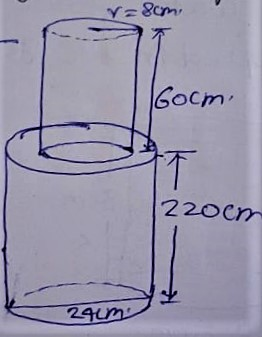Total Volume of iron pole = Volume of each cylindrical part

⇒ Total Volume of iron pole = πr²1h1 + πr²2h2

⇒ Total Volume of iron pole = π( r²1h1 + r²2h2 )

⇒ Total Volume of iron pole = π( 8×8×60 + 12×12×220 )

⇒ Total Volume of iron pole = π( 64×60 + 144×220 )

⇒ Total Volume of iron pole = π( 3840 + 31680 )

⇒ Total Volume of iron pole = 3.14×(35520)

⇒ Total Volume of iron pole = 111532.8 cm³

Mass of the Pole = 111532.8 × 8

⇒ Mass of the Pole = 892262.4 gm =892.2624kg

Q.7 A solid consisting of right circular cone of height 120 cm and radius 60 cm standing on a hemisphere of radius 60 cm is placed upright in a right circular cylinder full of water such as it touches the bottom. Find the volume of water left in the cylinder , if the radius of the cylinder is 60 cm and its height is 180 cm.

Given: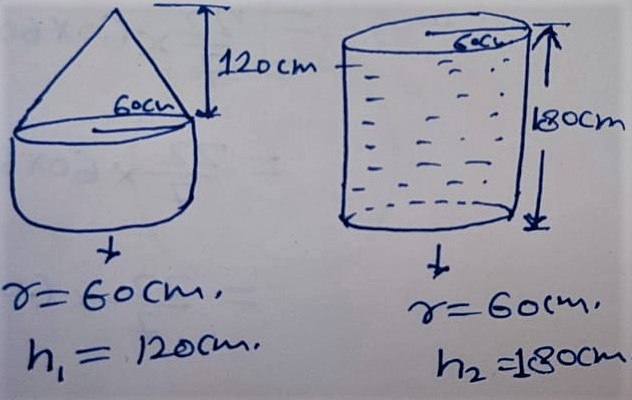To Find:

Volume of water left in the cylinder =?

Solution:

Volume of water left in the cylinder = Volume of cylinder – [ Volume of Cone + Volume of Hemisphere ]

⇒ Volume of water left in the cylinder = πr²h2 – ( 1/3 × πr²h1 + 2/3 × πr³ )

⇒ Volume of water left in the cylinder = πr²h2 – 1/3× πr²( h1 + 2×r )

⇒ Volume of water left in the cylinder = 22/7 × 60 × 60 [ 180 – 1/3× (120 + 2×60) ]

⇒ Volume of water left in the cylinder = 22/7 × 60 × 60 [ 180 – 1/3× (240) ]

⇒ Volume of water left in the cylinder = 22/7 × 60 × 60 [ 180 – 80 ]

⇒ Volume of water left in the cylinder = 22×360000 / 7

⇒ Volume of water left in the cylinder = 7920000 / 7

⇒ Volume of water left in the cylinder = 1131428.571 cm³

⇒ Volume of water left in the cylinder = 1.131428571 m³≈1.31 m³

Q.8 A Spherical glass vessel has a cylindrical neck 8 cm long, 2 cm in diameter, of the spherical part is 8.5 cm by measuring the amount of water it holds, a child finds its Volume to be 345 cm³. Check Whether she is correct, taking the above as the inside measurements , and π = 3.14.

Solution:

diameter = 2 cm

r = 2/2 = 1cm

h = 8 cm

Diameter of spherical part = 8.5 cm

r = 8.5/2

To Check:

Volume of vessel 345 cm³ …?

Solution: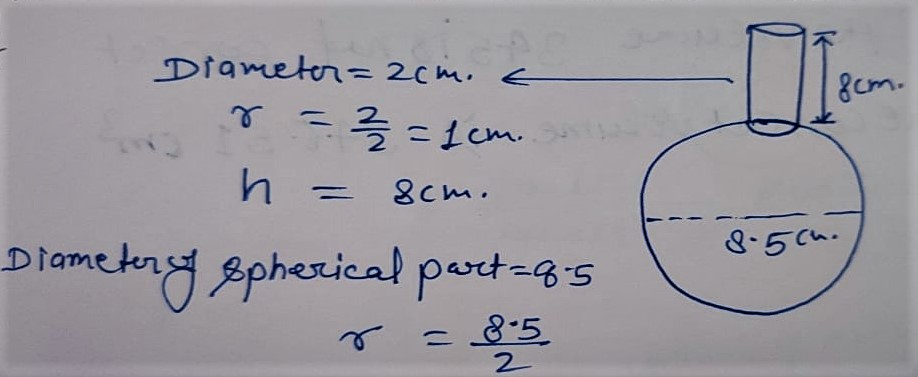Volume of vessel = Volume of spherical part + Volume of cylindrical part

⇒ Volume of vessel = 4/3 × πr³ + πr²h

⇒ Volume of vessel = π [ 4/3 × r³ + r²h ]

⇒ Volume of vessel = 3.14 [ 4/3 × 8.5/2 × 8.5/2 × 8.5/2 + 1²×8 ]

⇒ Volume of vessel = 3.14 [ 8.5×8.5×8.5/3×2 + 8 ]

⇒ Volume of vessel = 3.14 [ (614.125 + 48)/6 ]

⇒ Volume of vessel = 3.14 [ (662.125)/6 ]

⇒ Volume of vessel = (1.57×662.125)/3

⇒ Volume of vessel = 1039.53625/3

⇒ Volume of vessel = 346.512 cm³

Hence the Volume 345 cm³ is not correct The correct Volume is  346.512 cm³